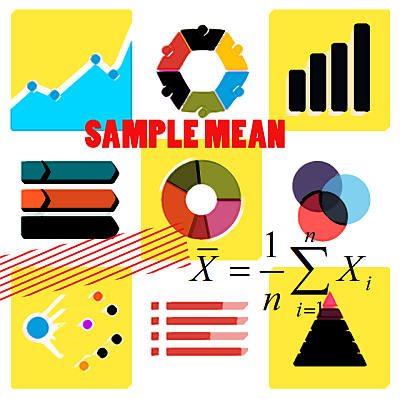# Sample Mean Calculator

Instructions: In order to use this sample mean calculator, please provide the sample data below and this solver will provide step-by-step calculation of the sample mean:Name of the variable (Optional)

The sample mean is one of the most commonly used measures of central tendency, that is used to summarize the data into one "average" value, that provides a measure of location of a distribution.

Let $$\{X_1, X_2, ..., X_n\}$$ be the sample data. The following formula is used to compute the sample mean:

$\bar X = \frac{1}{n}\sum_{i=1}^n X_i$

In other words, the sample mean is calculated by obtaining the sum of all the values in the sample , and then dividing by the number of elements in the sample.

Notice that the sample mean can be computed with Excel or other statistical calculator, but the advantage of this mean calculator is that it will show you all the steps.### Mean Calculation Example

For example, assume that the sample data is $$\{ 1, 2, 5, 8, 10\}$$, then, the sample mean is computed as follows:

$\bar X = \frac{1}{5}(1+2+5+8+10) = \frac{26}{5} = 5.2$

The sample mean is typically used as a representative measure of the center of the distribution. But, the problem with the sample mean is that it is too sensitive to extreme values. This indicates that when the distribution is significantly skewed the sample mean will tend to over-represent the skewed side.

In case of skewed distributions, it is recommended to use the sample median instead as the appropriate measure of central tendency. Or, if you interested in the dispersion of the data (as opposed to the measures of central tendency), this sample standard deviation calculator will help you

### The Sample Mean and other Descriptive Statistics

If you need to compute all the basic descriptive measures, including sample mean, variance, standard deviation , median, quartiles, etc, you can try our descriptive statistics calculator .

One crucial element to understand in Statistics is that the sample mean is in itself a random variable, and you compute probabilities associated to it. If that is what you need to do, using this probability of sample mean calculator

### What if I want to compute the population mean?

Observe that this is a sample mean calculator and not a population mean calculator. In order to compute the population mean, you will need to use the same formula, but you need to know ALL the data in the population (which sometimes could be hard to do for infinite populations).

### What if I have weights for each value?

If not all data carry the same weight, then the regular sample mean would not be appropriate, and you would need to use instead this weighted average calculator .